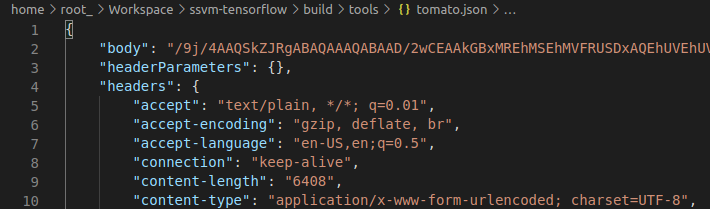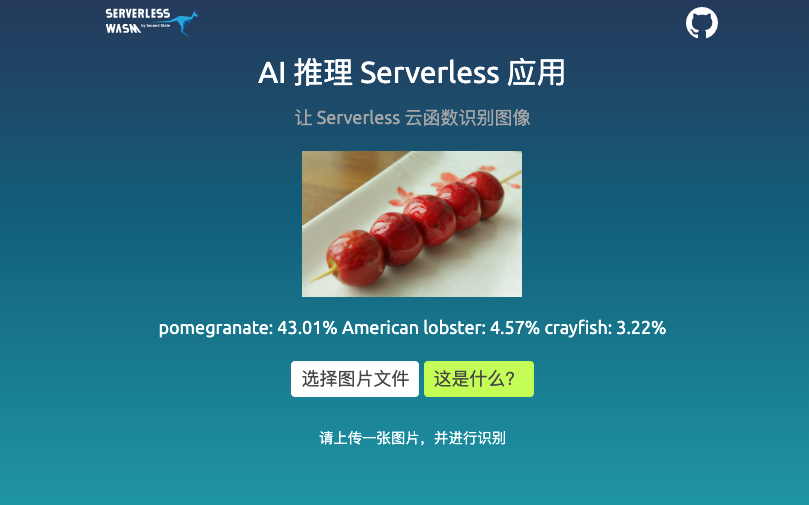# 用户体验测评笔记：OpenFaaS 和腾讯云 Serverless

## OpenFaaS

``````def handle(req):
print("Hello! You said: " + req)``````

``````version: 1.0
provider:
name: openfaas
gateway: http://127.0.0.1:8080
functions:
pycon:
lang: python3
handler: ./pycon
image: >>> dockerhub 用户名<<</pycon``````

OpenFaaS 提供一个叫 faas-cli 的部署工具，faas-cli 会先将镜像上传到相应的 docker hub 帐号名下，然后再下拉到 OpenFaaS 服务。

``````╰─➤  curl localhost:8080/function/pycon -d "Hi"
Hello! You said: Hi``````

1. 开发者只需要写好事件处理的函数，修改配置文件，确认部署即可，而不需要了解服务器的基础架构，甚至也不需要了解代码实际部署在哪个 Web 框架。
2. FaaS 服务返回调用接口。

## 将图像识别服务部署到腾讯云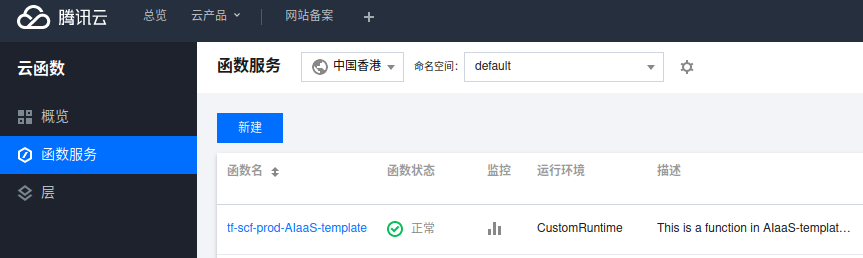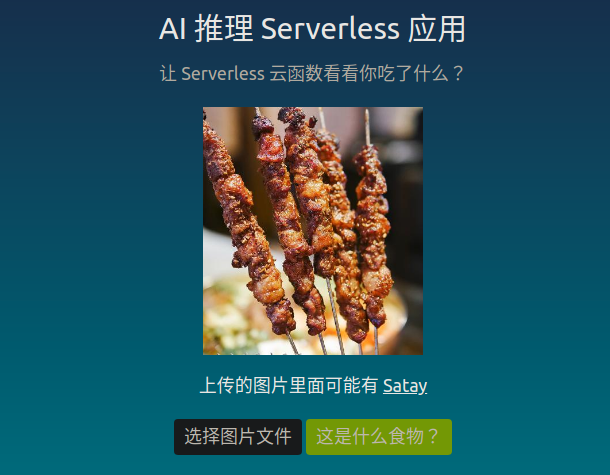## 魔改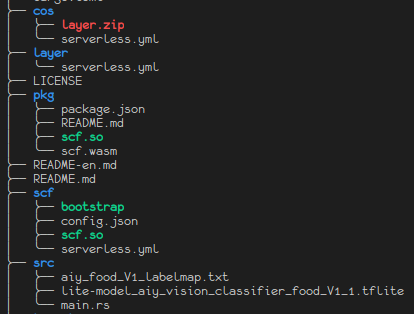cos, layer, scf 三个目录都有 serveress.yml，执行 `sls deploy` 的时候，可以看到这三个目录的文件被打包上传。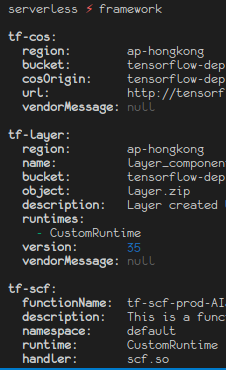`scf/bootstrap` 是一个脚本，运行后是一个服务进程。

``RESPONSE=\$(LD_LIBRARY_PATH=/opt /opt/ssvm-tensorflow "\$_HANDLER" <<< "\$EVENT_DATA")``

``````use std::io::{self, Read};
use ssvm_tensorflow_interface;
use serde::Deserialize;

fn search_vec(vector: &Vec<f32>, labels: &Vec<&str>, value: &f32) -> (i32, String) {
for (i, f) in vector.iter().enumerate() {
if f == value {
return (i as i32, labels[i].to_owned());
}
}
return (-1, "Unclassified".to_owned());
}

fn main() {
let model_data: &[u8] = include_bytes!("mobilenet_v2_1.4_224_frozen.pb");
let labels = include_str!("imagenet_slim_labels.txt");
let label_lines : Vec<&str> = labels.lines().collect();

let mut buffer = String::new();
let obj: FaasInput = serde_json::from_str(&buffer).unwrap();
let img_buf = base64::decode_config(&(obj.body), base64::STANDARD).unwrap();

let flat_img = ssvm_tensorflow_interface::load_jpg_image_to_rgb32f(&img_buf, 224, 224);

let mut session = ssvm_tensorflow_interface::Session::new(model_data, ssvm_tensorflow_interface::ModelType::TensorFlow);
session.add_input("input", &flat_img, &[1, 224, 224, 3])
.run();
let res_vec: Vec<f32> = session.get_output("MobilenetV2/Predictions/Softmax");
let mut sorted_vec = res_vec.clone();
sorted_vec.sort_by(|a, b| b.partial_cmp(a).unwrap());

let top1 = sorted_vec;
let top2 = sorted_vec;
let top3 = sorted_vec;

let r1 = search_vec(&res_vec, &label_lines, &top1);
let r2 = search_vec(&res_vec, &label_lines, &top2);
let r3 = search_vec(&res_vec, &label_lines, &top3);

println!("{}: {:.2}%\n{}: {:.2}%\n{}: {:.2}%"
, r1.1, top1 * 100.0
, r2.1, top2 * 100.0
, r3.1, top3 * 100.0
);
}

#[derive(Deserialize, Debug)]
struct FaasInput {
body: String
}``````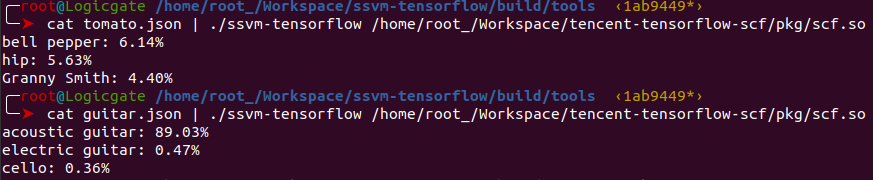tomato.json 用于模拟请求数据，将图片数据 base64 之后放在 "body" 后面。